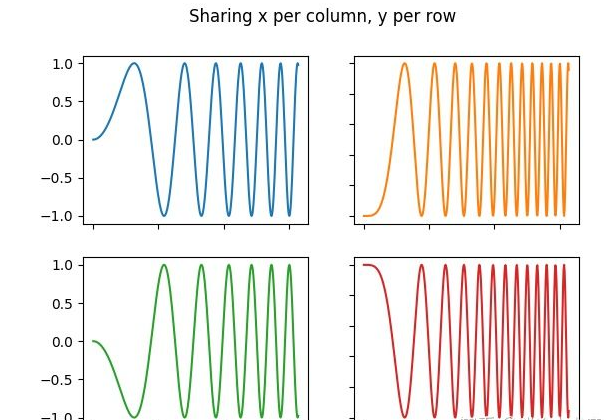• 写文章
• 提问

# python中如何用subplot画多个子图？Python可通过subplot函数画多个子图。调用形式如：subplot(nrows,ncols,index)，图表的整个绘图区域被分成nrows行和ncols列，按照从左到右，从上到下的顺序对每个子区域进行编号，左上的子区域编号为1。index参数指定创建的Axes对象所在的区域。

matplotlib官网上的一个2*2多子图例子如下所示：```import matplotlib.pyplot as plt
import numpy as np

# Some example data to display
x = np.linspace(0, 2 * np.pi, 400)
y = np.sin(x ** 2)

fig, ((ax1, ax2), (ax3, ax4)) = plt.subplots(2, 2)
fig.suptitle('Sharing x per column, y per row')
ax1.plot(x, y)
ax2.plot(x, y**2, 'tab:orange')
ax3.plot(x, -y, 'tab:green')
ax4.plot(x, -y**2, 'tab:red')

for ax in fig.get_axes():
ax.label_outer()```

```import matplotlib.pyplot as plt

plt.figure()
plt.subplot(211)
plt.plot(t1, Satarc)

plt.subplot(212)
plt.plot(t2, SNR)```